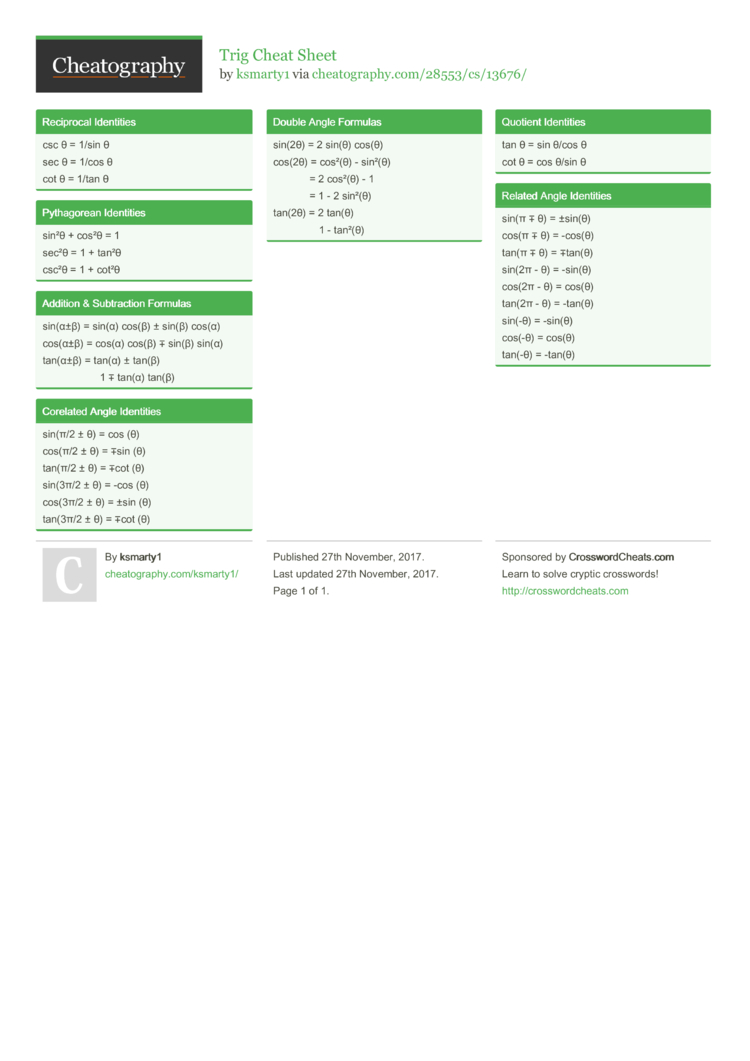Show Menu

# Trig Cheat Sheet by ksmarty1

### Reciprocal Identities

 csc θ = 1/sin θ sec θ = 1/cos θ cot θ = 1/tan θ

### Pythag­orean Identities

 sin²θ + cos²θ = 1 sec²θ = 1 + tan²θ csc²θ = 1 + cot²θ

### Addition & Subtra­ction Formulas

 sin(α±β) = sin(α) cos(β) ± sin(β) cos(α) cos(α±β) = cos(α) cos(β) ∓ sin(β) sin(α) tan(α±β) = tan(α) ± tan(β)       ­     1 ∓ tan(α) tan(β)

### Corelated Angle Identities

 sin(π/2 ± θ) = cos (θ) cos(π/2 ± θ) = ∓sin (θ) tan(π/2 ± θ) = ∓cot (θ) sin(3π/2 ± θ) = -cos (θ) cos(3π/2 ± θ) = ±sin (θ) tan(3π/2 ± θ) = ∓cot (θ)

### Double Angle Formulas

 sin(2θ) = 2 sin(θ) cos(θ) cos(2θ) = cos²(θ) - sin²(θ)       ­   ­   ­   ­   ­   ­   ­   ­   ­   ­   ­   ­   ­   ­   ­   ­   ­   ­   ­   ­   ­    = 2 cos²(θ) - 1       ­   ­   ­   ­   ­   ­   ­   ­   ­   ­   ­   ­   ­   ­   ­   ­   ­   ­   ­   ­   ­    = 1 - 2 sin²(θ) tan(2θ) = 2 tan(θ)       ­   ­   ­   ­   ­   ­   ­   ­   ­   ­   ­   ­   ­   ­   ­   ­   ­   ­   ­   ­   ­   ­   ­   ­   ­   ­   ­    1 - tan²(θ)

### Quotient Identities

 tan θ = sin θ/cos θ cot θ = cos θ/sin θ

### Related Angle Identities

 sin(π ∓ θ) = ±sin(θ) cos(π ∓ θ) = -cos(θ) tan(π ∓ θ) = ∓tan(θ) sin(2π - θ) = -sin(θ) cos(2π - θ) = cos(θ) tan(2π - θ) = -tan(θ) sin(-θ) = -sin(θ) cos(-θ) = cos(θ) tan(-θ) = -tan(θ)

## Download the Trig Cheat Sheet1 Page
//media.cheatography.com/storage/thumb/ksmarty1_trig.750.jpg

PDF (recommended)

Alternative Downloads

## Comments

No comments yet. Add yours below!

## Add a Comment

Your Comment

Please enter your name.

Please enter your email address

Please enter your Comment.

## Related Cheat Sheets

Discrete Math Cheat Sheet
JavaScript Object Methods Cheat Sheet
Linear Algebra Cheat Sheet